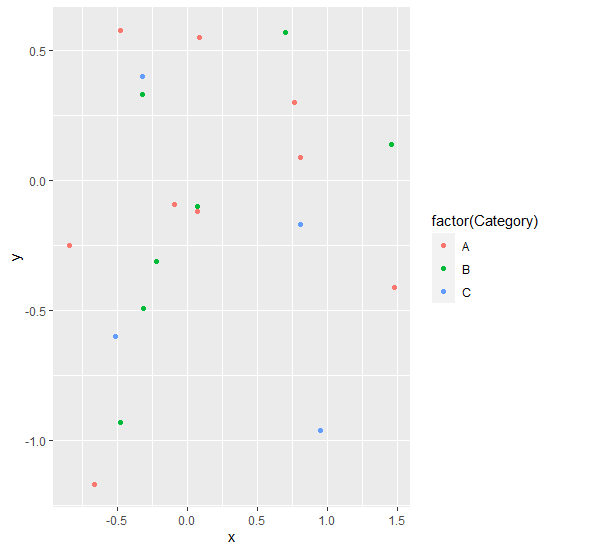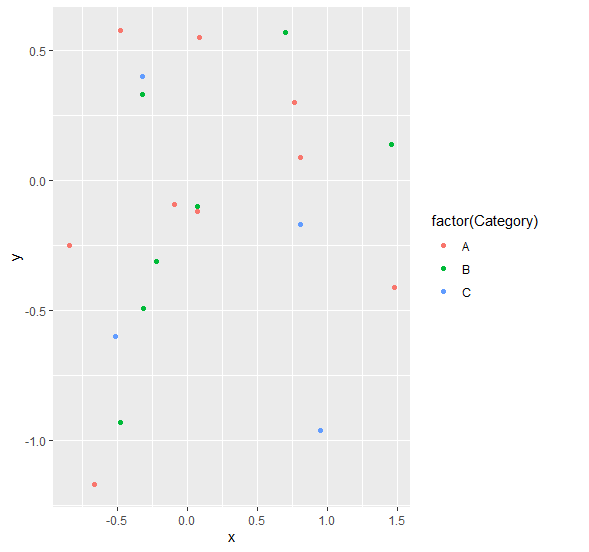# Remove grey color from legend display using ggplot2 in R.

R ProgrammingServer Side ProgrammingProgramming

To remove grey color from legend display using ggplot2, we can use theme function where we can fill legend.key argument to white with element_rect.

For Example, if we have a data frame called df that contains three columns say X and Y and F where X and Y are numerical and F is categorical then we can create scatterplot between X and Y without grey color in legend display by using the command given below −

ggplot(df,aes(X,Y))+geom_point(aes(colour=factor(F)))+theme(legend.key=element_rect(fill="white"))

## Example

Following snippet creates a sample data frame −

x<-round(rnorm(20),2)
y<-round(rnorm(20),2)
Category<-sample(LETTERS[1:3],20,replace=TRUE)
df<-data.frame(x,y,Category)
df

The following dataframe is created

      x     y  Category
1 -0.22 -0.31  B
2  0.81  0.09  A
3  0.81 -0.17  C
4 -0.09 -0.09  A
5  0.70  0.57  B
6 -0.48 -0.93  B
7  1.46  0.14  B
8  0.07 -0.12  A
9 -0.32  0.40  C
10  0.95 -0.96  C
11 -0.32  0.33  B
12 -0.84 -0.25  A
13  0.07 -0.10  B
14 -0.48  0.58  A
15 -0.51 -0.60  C
16  1.48 -0.41  A
17 -0.66 -1.17  A
18 -0.31 -0.49  B
19  0.77  0.30  A
20  0.09  0.55  A

To load ggplot2 package and create scatterplot between x and y with color of points based on Category column on the above created data frame, add the following code to the above snippet −

x<-round(rnorm(20),2)
y<-round(rnorm(20),2)
Category<-sample(LETTERS[1:3],20,replace=TRUE)
df<-data.frame(x,y,Category)
library(ggplot2)
ggplot(df,aes(x,y))+geom_point(aes(colour=factor(Category)))

## Output

If you execute all the above given snippets as a single program, it generates the following Output −To create scatterplot between x and y with color of points based on Category column without grey color in legend display on the above created data frame, add the following code to the above snippet −

x<-round(rnorm(20),2)
y<-round(rnorm(20),2)
Category<-sample(LETTERS[1:3],20,replace=TRUE)
df<-data.frame(x,y,Category)
library(ggplot2)
ggplot(df,aes(x,y))+geom_point(aes(colour=factor(Category)))+theme(legend.key=element_rect(fill="white"))

## Output

If you execute all the above given snippets as a single program, it generates the following Output −